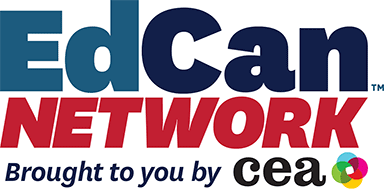EdTech & Design

# Using EquatIO® to Support Digital Math Instruction

For years, one of the most common questions that I heard as an educational technology trainer, speaker, and coach was “I’m a math teacher. How can I use edtech in my class?”

I had lots of answers that I was excited about. I advocated for Desmos activities, Flipgrid topics for sharing strategies, spreadsheets for investigating patterns, and more. One thing that was always tough, though, was actually entering mathematical representations into digital spaces. Some spaces were built for it, like Desmos, and some spaces had a pen tool, like Flipgrid, but others were not set up well for it, like Google Docs, Slides, and Forms.

Until EquatIO® came along. With its “Make math digital” tagline, Texthelp’s tool gave teachers and students the ability to easily enter equations and graphs into Docs, Slides, Forms, and more. At that time, there were quite a few math and science teachers who were very excited about the capabilities that EquatIO gave them. They enjoyed using them to create content, activities, and assignments for their students. And, for some of them, they even had their students use EquatIO to respond or create content of their own.

From my observations, some math teachers may have thought that EquatIO was a misspelling of equation. Until early 2020, that is. When math classes, along with all of the other classes in schools, moved online, educators needed a way to create, as EquatIO calls it, “Make math digital.”

And I think that EquatIO is one tool that they should consider to support their digital math instruction.

EquatIO–which is free for teachers–has 8 main features that I’d like to share with you.  The first 5 relate to entering math and science expressions into digital spaces. Let’s look at those first.

Entering Math and Science Expressions with EquatIONote that while this EduGIF shows EquatIO being used in Google Forms, it also works in Slides, Docs, Sheets, and Drawings, as well as Microsoft Word, Microsoft PowerPoint, and Office 365 applications.

EQUATION EDITOR

As you can see above, the equation editor lets you type in words, numbers, letters, and symbols which it then turns into equations or expressions.

LATEX EDITOR

If you don’t know what LaTeX is (yes, that’s the way it’s really capitalized), don’t worry: that’s why EquatIO exists. You’ve been able to use LaTeX to enter mathematical expressions for decades, but most educators, myself included, didn’t (and don’t) know how. And that’s okay, because EquatIO gives us a bunch of other options. But if you know how to use LaTeX, EquatIO has that for ya, too.

HANDWRITING RECOGNITION

You draw a pi symbol, EquatIO turns it into π. You draw a square root, EquatIO turns it into √ . Cube roots, arcs, absolute values, Greek symbols, and logic symbols, too! You draw the math, EquatIO turns it into digital math!

SPEECH INPUT

You say pi, EquatIO turns it into π. You say square root, EquatIO turns it into √ . Yup! You say the math, EquatIO turns it into digital math!

EQUATIO MOBILE

You can also use your mobile device to prepare, capture, or create your mathematical expressions! Just access the EquatIO site from your mobile device with your normal EquatIO account and you’ll be able to use speech input, handwriting recognition, or even the camera to send content–as text or images–straight to your computer! You can learn more about this feature here.

What else can you do with EquatIO?

EquatIO has more to offer to your math or science classroom than just a set of awesome modes for entering expressions and equations. Let’s check out the other features:

GRAPH EDITOR

You’re most likely familiar with the Desmos graphing calculator. Along with their scientific calculator, it is “used annually by over 40 million teachers and students around the world.” They are “built into the majority of U.S. state-level assessments and digital college entrance exams” and a number of other assessments. (desmos.com/testing) Well, it’s built into EquatIO, too.  Want to give your students a graph to answer questions about? Want to give them an equation and ask them to pick the correct graph from a set of choices? Want them to respond to a question with a graphical representation? You can do it all using the Graph Editor in EquatIO.  Enter your equation and then insert the graph right into your Forms, Docs, Slides, or Sheets!